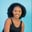Related Tags

python3
numpy

# How to use the numpy.conjugate() method for a 2-D arrayMaria Elijah

## The numpy.conjugate() method

The numpy.conjugate() method returns a complex conjugate of an input array that is passed to it. This is done element by element.

Note: In Python, we can use a list of lists to create a two-dimensional (2-D) array.

### Syntax

numpy.conjugate(arr, /, out=None, *, where=True,)


### Parameters

• arr1: This represents the input array.
• out: This optional parameter specifies the location where the result is saved.
• where: This is an optional parameter representing the condition in which the input gets broadcasted.

### Return value

The method numpy.conjugate() returns the complex element-wise conjugate of the input array passed to it.

### Example

The following code shows how to use the numpy.conjugate() method for two-dimensional (2-D) arrays.

# import numpy
import numpy as np
# create list
x1 = [1+2j,1+5j,1+3j]
x2 = [1+7j,1+2j,1+5j]
# convert the lists to 2D array using np.array
arr = np.array([x1,x2])

# compute the complex array values
# and store the result in result
result = np.conjugate(arr)

print(result)
Using the np.conjugate() function

### Explanation

• Line 2: We add the numpy library to the code.
• Lines 4–5: We create two lists, x1 and x2.
• Line 7: We use the np.array() method to turn the lists into a 2-D array and save the result in a new variable named arr.
• Line 11: We compute the input array’s complex conjugate using the np.conjugate() method and store the result in the result variable.
• Line 13: We display the output.

RELATED TAGS

python3
numpy

CONTRIBUTORMaria Elijah
RELATED COURSES

View all Courses

Keep Exploring

Learn in-demand tech skills in half the time